# No Load Losses in Transformer

## What are No-Load Losses( Excitation Losses)?

Transformer no load losses occur when the transformer is excited at the rated voltage and frequency keeping its secondary open circuit. Transformer no-load losses are combined losses caused by eddy current loss, hysteresis loss, stray eddy current loss, and dielectric loss. The maximum losses when the transformer is under no load occur in the core. Therefore, the No-Load losses of the transformer are also called iron loss or excitation loss. Transformer no-load losses are constant from no load to full load, therefore no-load losses are also called constant losses.
A transformer is a static device, and there are no moving parts in the transformer. Therefore, the friction and windage losses in the transformer are nil. The transformer steps up or steps down the voltage keeping the frequency unaltered. Transformer losses occur because of current flowing in the winding and magnetic field in the core.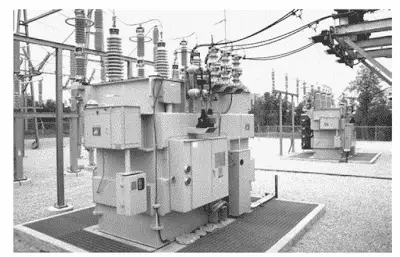For an ideal transformer, the input power is equal to output power, however, in reality, this is not true. The output power of the transformer is always less than the input power. The energy can neither be created, nor it can be destroyed; the same principle is applicable for the energy balancing of the transformer as well.

The power drawn at the primary is equal to the losses in the transformer plus the power delivered at the secondary side of the transformer. The losses in the transformer can be broadly categorized into two categories;
1. Iron losses or core losses, dielectric loss, and stray eddy current loss

2. Copper loss and Stray losses

## Iron losses or core losses:

The voltage applied at the primary winding produces a magnetic field in the core. The flux produced due to primary current passes through the core, and it gets linked to the secondary winding of the transformer and induces a voltage in the secondary of the transformer.
In this induction process when the transformer is at no load, the transformer suffers from iron or core losses that can be further categorized into two categories viz. hysteresis loss, eddy current loss, stray eddy current loss, and dielectric loss. These all losses are constant and therefore the no-load losses are also called constant loss of the transformer.

## Hysteresis Loss

##### What is hysteresis loss in the transformer?
When the voltage is applied to the primary of the transformer, the alternating current flowing in the primary magnetizes the core. The core goes under the magnetization and demagnetization process because of the flow of current in the forward and reverses direction. With the reversal of the direction of the alternating current, the energy is lost in the form of heat in the core. The heat loss due to the repeated reversal of the magnetic field in the core is known as hysteresis loss.

The hysteresis loss can be calculated by finding the area of the B-H curve. To minimize hysteresis loss, the core of the transformer is made of Cold Rolled Grain Oriented (CRGO) Silicon Steel because the magnetization curve area of this material is less compared to other magnetic materials.

###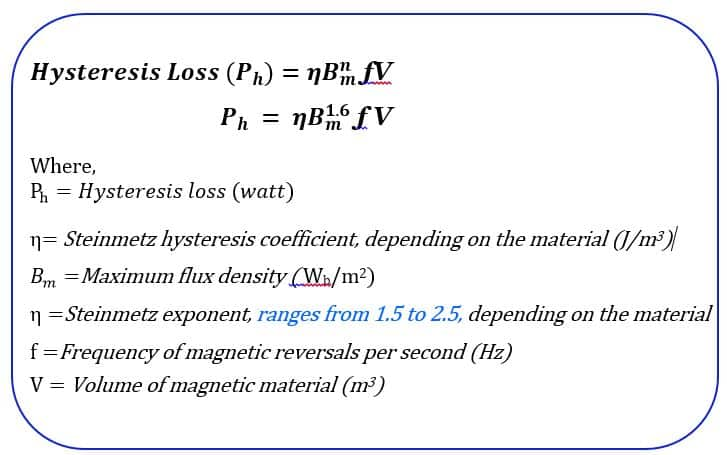Eddy Current Loss

#### What is Eddy Current loss in the transformer?

The flux that passes through the core induces a voltage of different magnitude at various places on the surface of the core and the other conducting parts of the transformer. Because of potential difference at various places on the core surface, the current set up in the core. These current set up in the core is called eddy currents.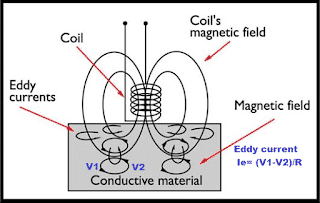Because of eddy currents, the heat loss equal to  I2R  loss occurs in the core.

The eddy current loss depends on the square of the current flowing in the upper part of the core and the resistance of the core material. The solid sheet of the iron block has less resistance because of the larger cross-section area. The magnitude of the voltage induced in a solid block is more and as a result, the eddy current loss is more in the solid iron block.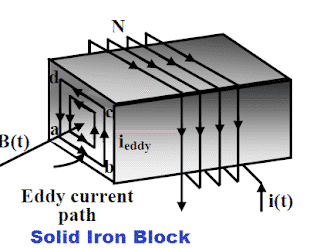### Why is the iron core of a transformer Laminated?

To minimize the eddy current losses, the iron core is formed using several thin electrically insulated sheets called lamination.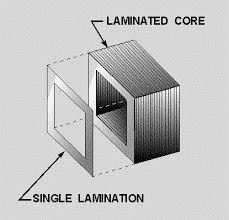The area of the eddy current path gets reduced and the losses decrease because of the decrease of induced voltage and increase of resistance. The thin laminated sheet has higher resistance. The flow of eddy current in laminated sheets is as given below.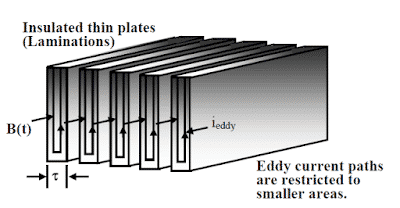The current flowing in the sheet is equal to;

I= Potential difference of the EMF induced in laminated core/Resistance of the sheet.

As the thin sheet has higher resistance as compared to the resistance of the thick sheet, the current flowing through the thin sheet is very low in comparison to the current flowing through the thick sheet. With the reduction in the magnitude of the eddy current in thin stamping, the eddy current loss reduces drastically. That is why the core of the transformer is made of thin laminated sheets.

In addition to the dependency of eddy current on the stamping resistance, other electrical parameters like primary voltage and frequency also affect the eddy current loss.

The eddy current loss formula of the transformer is given below.

#### Eddy Current Loss Formula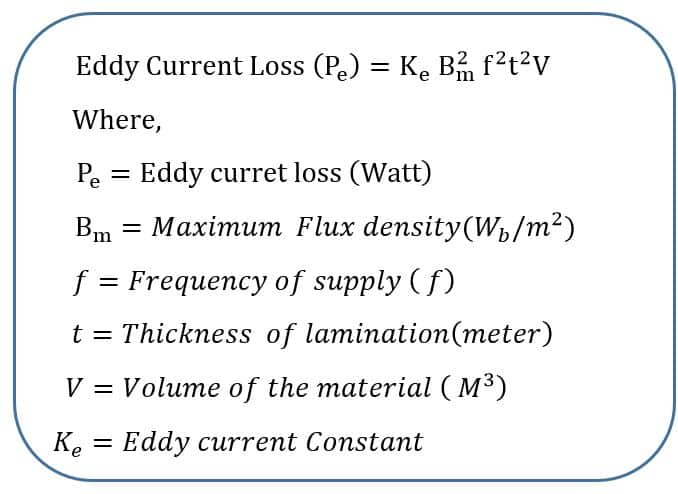### Effect of Voltage and Frequency Variation on Transformer

The transformer is designed for particular voltage and frequency. The change in supply voltage and frequency from the designed value will further lead to an increase in eddy current loss. The transformer manufacturer gives a guarantee for iron loss for the operation of the transformer at rated voltage and frequency.
If the system voltage increases, the maximum flux density in the core increases, and consequently the eddy current loss and hysteresis loss increase. To protect the transformer from over fluxing, V/F ratio is measured and the transformer can be switched off if the flux in the core reaches above the specified flux limit of the transformer.
The eddy current losses drastically increase with an increase in the frequency if the voltage is also increased in the same proportion because the eddy current loss is proportional to the square of the frequency.
Generally, system frequency remains within the limit of rated frequency +/- 1.5 Hz; however, if the electrical network rich in harmonics can lead to an increase in the eddy current loss. With an increase in the frequency, the eddy current loss increases much more than the hysteresis loss because the eddy current loss is proportional to the square of the frequency, whereas the hysteresis loss is proportional to the frequency.
Thus the no-load losses of the transformer are equal to the sum of the eddy current loss and the hysteresis loss.
The no-load losses of the transformer are constant for a rated voltage and frequency. Therefore, the no-load loss is also called a constant loss. The no-load losses changes if the transformer is operated above its rated flux density. Moreover, the increased flux density cause distorted secondary output voltage, and the transformer if operated above the rated flux density, it is apt to fail. Therefore, over fluxing protection is employed for the protection of the transformer.
Variation in no-load losses with variation in voltage and or frequency

Let us understand how the hysteresis and eddy current loss get affected by changes in frequency and voltage. We will take the four cases for the study of no-load losses of transformer.

##### Case 1Effect on no-load losses – When the frequency is increased/decreased keeping voltage constant

The hysteresis loss is proportional to the frequency. The hysteresis loss should increase with an increase in the frequency, however, the hysteresis loss remains almost unchanged. The reason is that the flux density in the core gets decreased in the same proportion of the increased frequency.

In a similar way, the hysteresis loss should decrease with a decrease in frequency, however, the hysteresis loss remains almost unchanged because of increased flux density in the core.

The eddy current loss is proportional to the square of the flux density and frequency. With an increase in the frequency, the eddy current remains unchanged because the product of B2m f2 remains unchanged as flux is proportional to the ratio of V/f. With the decrease in frequency, the flux density in the core gets increased in the same proportion of frequency decrease and thus the eddy current loss remains unchanged.

##### Case 2Effect on no-load losses- When the voltage is increased/decreased keeping the frequency constant

The hysteresis loss is directly proportional to the voltage and flux density. The hysteresis loss increase with an increase in voltage. The magnetic flux density is also proportional to the voltage. Thus the hysteresis loss is proportional to the square of voltage if the frequency is kept constant.
Thus,
Hysteresis loss, Wh ∝ V2
Eddy current loss, We ∝ V3

##### Case 3Effect on no-load losses – When the frequency is increased/decreased and voltage is also increased/decreased in the same proportion

If the frequency is increased and voltage is also increased in the same proportion then the flux density in the core remains unchanged and, in this case, the hysteresis loss will increase proportionally to the increase of frequency, The eddy current loss will increase in the square proportion of the increased frequency.

##### Case 4Effect on no-load losses – When the frequency is increased/decreased and voltage is also increased/decreased in different proportion

In this situation, the eddy current and hysteresis loss will increase or decrease because of the following reasons.

• Increase/decrease of frequency
• Increase/decrease of flux density

Related Post

### 4 thoughts on “No Load Losses in Transformer”

1.Scales are very essential device or equipment which helps you to measure the objects in a very precise manner. This provides you with the exact measurement of the things which are produced or manufactured. load cell

Reply